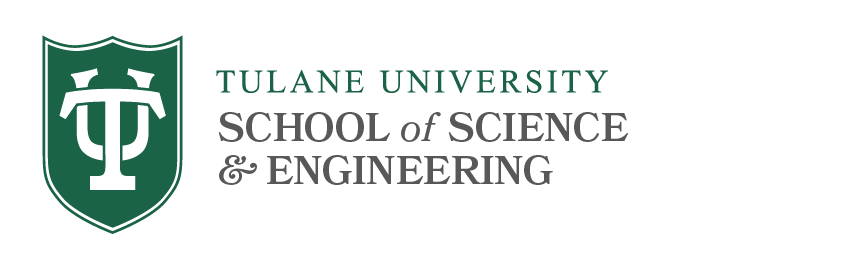# 4000 Level Courses

Math 4060 Real Analysis II (3)
Prerequisites: Math 3050 and 3090.
An in-depth treatment of multivariable calculus. Extends the material covered in Mathematics 2210. Chain rule, inverse and implicit function theorems, Riemann integration in Euclidean n-space, Gauss-Green-Stokes theorems, applications.

Math 4120 Abstract Algebra II (3)
Prerequisites: Math 3090 and 3110.
Abstract vector spaces, quotient spaces, linear transformations, dual spaces, determinants. Solvable groups. Field extensions, Galois theory, solvability of equations by radicals.

Math 4210/6210 Differential Geometry (3)
Prerequisites: Math 3050 and 3090.
Theory of plane and space curves including arc length, curvature, torsion, Frenet equations, surfaces in three-dimensional space. First and second fundamental forms, Gaussian and mean curvature, differentiable mappings of surfaces, curves on a surface, special surfaces.

Math 4240/6240 Ordinary Differential Equations (3)
Prerequisites: Math 3090.
Review of linear algebra, first-order equations (models, existence, uniqueness, Euler method, phase line, stability of equilibria), higher-order linear equations, Laplace transforms and applications, power series of solutions, linear first-order, systems (autonomous systems, phase plane), application of matrix normal forms, linearization and stability of nonlinear systems, bifurcation, Hopf bifurcation, limit cycles, Poincare-Bendixson theorem, partial differential equations (symmetric boundary-value problems on an interval, eigenvalue problems, eigenfunction expansion, initial-value problems in 1D).  Students may not receive credit for both 2240 and 4240.

Math 4250/6250 Mathematical Foundations of Computer Security (3)
Prerequisites: Calculus, Math 2170 and Math 3110 or the permission of the instructor.
This course studies the mathematics underlying computer security, including both public key and symmetric key cryptography, crypto-protocols and information flow. The course includes a study of the RSA encryption scheme, stream and clock ciphers, digital signatures and authentication. It also considers semantic security and an analysis of secure information flow.

Math 4300/6300 Complex Analysis (3)
Prerequisite: Math 3050.
The complex number system, complex integration and differentiation, conformal mapping, Cauchy’s theorem, calculus of residues.

Math 4410 Topology (3)
Prerequisite: Math 3050.
An introduction to topology. Elementary point set topology: topological spaces, compactness, connectedness, continuity, homeomorphisms, product and quotient spaces. Classification of surfaces and other geometric applications.

Math 4470/6470 Analytical Methods of Applied Mathematics (3)
Prerequisites: Math 2210 and 2240.
Derivations of transport, heat/reaction-diffusion, wave. Poisson's equations; well-posedness; characteristics for first order PDE's; D'Alembert formula and conservation of energy for wave equations; propagation of waves; Fourier transforms; heat kernel, smoothing effect; maximum principles; Fourier series and Sturm-Liouville eigenexpansions; method of separation of variables; frequencies of wave equations, stable and unstable modes, long-time behavior of heat equations; delta function; fundamental solution of Laplace equation, Newton potential; Green's function and Poisson formula; Dirichlet Principle.

Math 4780 Introduction to Concurrency (3)
Prerequisites: Math 2170 and Math 3100 or approval of instructor.
This course is a general introduction to Concurrency, i.e., the Mathematical modeling of systems made up of several processes interacting with each other. The process algebra CSP (Communicating Sequential Processes) will be studied, both on the syntactic and semantic level. The denotational, operational, and algebraic models used to reason about the language will be presented, and examples will be used throughout to illustrate the theory.

Math 4900 (3)
Prerequisite: Permission of the Instructor.
This course covers a variety of advances in topics in mathematics and exposes students to recent developments not available in other parts of the mathematics curriculum. It meets ini conjunction with graduate level courses MATH 7710-7790 Topics covered will vary from semester to semester. Recent topics offered include Knot Theory and 3-Manifolds, Algebraic Combinatorics, Cardiac Modeling, Number Theory. Students may receive credit for MATH 490 more than once, when the topics covered are distinct. Each section will have the specific topic listed as a subtitle and will have specific prerequisites to the 300 level or above.

Math 4910, 4920 Independent Studies (1-3, 1-3)
Prerequisite: Approval of the department.
No more than four hours of 4910-4920 may be counted toward satisfying the major requirements.

Math H4990-H5000 Honors Thesis (3, 4)
Prerequisite: Approval of the department.
Thesis may serve to satisfy part of the departmental honors requirements.